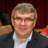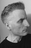calculate difference between field values in current and previous record

Hi,

Calculating a field value from 2 other fields in the same record is straightforward.
Now I'm trying to create a formula field that calulates the difference between the value of a field in the current record and the value of the same field in the previous record.

How do I go about that?

It concerns a list of dates and measurement values. Each time a new record is created, I want the difference with the previous measurement to be calculated automatically. Preferably the previous record is not the previous record ID but chronologically the previous record date.

Thanks!

LievenHi Lieven,

as a formula field:

---

let myRec := this;
let prevDate := max((select 'Your Table')[Date < myRec.Date].Date);
if prevDate then
let prevRec := first((select 'Your Table')[Date = prevDate]);
myRec.Value - prevRec.Value
else
0
end

---

Leo

Is there also a possibility to calculate the value of the former row. I'm working on a database for kilometer calculation. Each row contains the data for one week. When I enter a new row, I would like to see the amount of kilometers in the former row (past week) calculated. HansHi Hans,

If you write the weeks as numbers (32,33,34 ...) then you can do it like this:

---

let myRec := this;
let lastWeek := max((select 'Your Table')[Week < myRec.Date].Week);
if lastWeek then
let prevRec := first((select 'Your Table')[Week = lastWeek]);
prevRec.'KILOMETER FIELD'
else
0
end

---

Leo

Hans@Leonid Semik, thank you for sharing this formula, but I am having trouble with it in Ninox Cloud in my Journal table with over 10K records, as the table view just hangs and shows no records at all. I had to delete the formula field "DURATION". Any ideas?

Example:

Id       JournalNo Date            DaysSince  DURATION

75619 1             2006-11-07  4,750

75620 2             2006-11-12  4,745         5

75621 3             2006-12-01  4,726         19

75622 4             2006-12-07  4,720         6